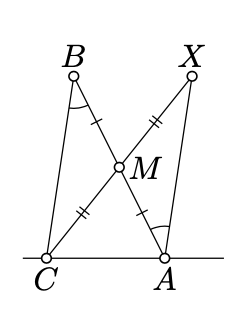$$\newcommand{\id}{\mathrm{id}}$$ $$\newcommand{\Span}{\mathrm{span}}$$ $$\newcommand{\kernel}{\mathrm{null}\,}$$ $$\newcommand{\range}{\mathrm{range}\,}$$ $$\newcommand{\RealPart}{\mathrm{Re}}$$ $$\newcommand{\ImaginaryPart}{\mathrm{Im}}$$ $$\newcommand{\Argument}{\mathrm{Arg}}$$ $$\newcommand{\norm}{\| #1 \|}$$ $$\newcommand{\inner}{\langle #1, #2 \rangle}$$ $$\newcommand{\Span}{\mathrm{span}}$$

# 11.2: Two angles of a triangle

$$\newcommand{\vecs}{\overset { \rightharpoonup} {\mathbf{#1}} }$$ $$\newcommand{\vecd}{\overset{-\!-\!\rightharpoonup}{\vphantom{a}\smash {#1}}}$$$$\newcommand{\id}{\mathrm{id}}$$ $$\newcommand{\Span}{\mathrm{span}}$$ $$\newcommand{\kernel}{\mathrm{null}\,}$$ $$\newcommand{\range}{\mathrm{range}\,}$$ $$\newcommand{\RealPart}{\mathrm{Re}}$$ $$\newcommand{\ImaginaryPart}{\mathrm{Im}}$$ $$\newcommand{\Argument}{\mathrm{Arg}}$$ $$\newcommand{\norm}{\| #1 \|}$$ $$\newcommand{\inner}{\langle #1, #2 \rangle}$$ $$\newcommand{\Span}{\mathrm{span}}$$ $$\newcommand{\id}{\mathrm{id}}$$ $$\newcommand{\Span}{\mathrm{span}}$$ $$\newcommand{\kernel}{\mathrm{null}\,}$$ $$\newcommand{\range}{\mathrm{range}\,}$$ $$\newcommand{\RealPart}{\mathrm{Re}}$$ $$\newcommand{\ImaginaryPart}{\mathrm{Im}}$$ $$\newcommand{\Argument}{\mathrm{Arg}}$$ $$\newcommand{\norm}{\| #1 \|}$$ $$\newcommand{\inner}{\langle #1, #2 \rangle}$$ $$\newcommand{\Span}{\mathrm{span}}$$

In this section we will prove a weaker form of Theorem 7.4.1 which holds in any neutral plane.

Theorem $$\PageIndex{1}$$

Let $$\triangle ABC$$ be a nondegenerate triangle in the neutral plane. Then

$$|\measuredangle CAB| + |\measuredangle ABC| < \pi.$$

Note that according to Theorem 3.3.1, the angles $$ABC, BCA$$, and $$CAB$$ have the same sign. Therefore, in the Euclidean plane the theorem follows immediately from Theorem 7.4.1.

ProofLet $$X$$ be the reflection of $$C$$ across the midpoint $$M$$ of $$[AB]$$. By Proposition 7.2.1 $$\measuredangle BAX = \measuredangle ABC$$ and therefore

$\measuredangle CAX \equiv \measuredangle CAB + \measuredangle ABC.$

Since $$[BM]$$ and $$[MX]$$ do not intersect $$(CA)$$, the points $$B$$, $$M$$, and $$X$$ lie on the same side of $$(CA)$$. Therefore the angles $$CAB$$ and $$CAX$$ have the same sign. By Theorem 3.3.1, the angles $$CAB, ABC$$ have the same sign; that is all angles in 11.2.1 have the same sign.

Note that $$\measuredangle CAX \not\equiv \pi$$, otherwise $$X$$ would lie on $$(AC)$$. Therefore the identity 11.2.1 implies that

$$|\measuredangle CAB|+|\measuredangle ABC|=|\measuredangle CAX| < \pi.$$

Exercise $$\PageIndex{1}$$

Assume $$A,B,C$$, and $$D$$ are points in a neutral plane such that

$$2 \cdot \measuredangle ABC + 2 \cdot \measuredangle BCD \equiv 0.$$

Show that $$(AB)\parallel (CD)$$.

Note that one cannot apply the transversal property (Theorem 7.3.1)

Hint

Arguing by contradiction, assume $$2 \cdot (\measuredangle ABC + \measuredangle BCD) \equiv 0$$, but $$(AB) \nparallel (CD)$$. Let $$Z$$ be the point of intersection of $$(AB)$$ and $$(CD)$$.

Note that $$2 \cdot \measuredangle ABC \equiv 2 \cdot \measuredangle ZBC$$, and $$2 \cdot \measuredangle BCD \equiv 2 \cdot \measuredangle BCZ$$.

Apply Proposition $$\PageIndex{1}$$ to $$\triangle ZBC$$ and try to arrive at a contradiction.

Exercise $$\PageIndex{2}$$

Prove the side-angle-angle congruence condition in the neutral geometry.

In other words, let $$ABC$$ and $$A'B'C'$$ be two triangles in a neutral plane; suppose that $$\triangle A'B'C'$$ is nondegenerate. Show that $$\triangle ABC \cong \triangle A'B'C'$$ if

$$AB = A'B'$$,     $$\measuredangle ABC = \pm \measuredangle A'B'C'$$     and     $$\measuredangle BCA = \pm \measuredangle B'C'A'.$$

HintLet $$C'' \in [B'C')$$ be the point such that $$B'C'' = BC$$.

Note that by SAS, $$\triangle ABC \cong \triangle A'B'C''$$. Conclude that $$\measuredangle B'C'A' = \measuredangle B'C''A'$$.

Therefore, it is sufficient to show that $$C'' = C'$$. If $$C' \ne C''$$ apply Proposition $$\PageIndex{1}$$ to $$\triangle A'C'C''$$ and try to arrive at a contradiction.

Note that in the Euclidean plane, the above exercise follows from ASA and the theorem on the sum of angles of a triangle (Theorem 7.4.1). However, Theorem 7.4.1 cannot be used here, since its proof uses Axiom V. Later (Theorem 13.1.1) we will show that Theorem 7.4.1 does not hold in a neutral plane.

Exercise $$\PageIndex{3}$$

Assume that the point $$D$$ lies between the vertices $$A$$ and $$B$$ of $$\triangle ABC$$ in a neutral plane. Show that

$$CD < CA$$     or      $$CD < CB$$.

Hint

Use Exercise 5.2.2 and Proposition $$\PageIndex{1}$$.

Alternatively, use the same argument as in the solution of Exercise 5.6.1.